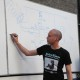## Example code from presentation: Ways of Seeing Julia Sets

I gave a presentation at yesterday’s DIT School of Mathematical Sciences lunchtime research seminar titled Ways of Seeing Julia Sets: Visualizing the forces that shape fractal Julia sets. This was the abstract:

Early in the 20th century, work by mathematicians such as Pierre Fatou and Gaston Julia on complex dynamics led to the definition of so-called Julia sets. When a rational complex polynomial function is applied iteratively to a complex number, z, it produces a sequence of complex values called the orbit of z. Depending on the particular function and on the value of z, that orbit may or may not remain bounded. For a given function, the Julia set forms the boundary between those regions of the complex plane where the orbits remain bounded and those where they do not.Intriguingly, even for quite simple iterative complex functions, Julia sets often take on very striking fractal shapes. With the aid of a computer, it is easy to visualize the Julia set of a function but, for most people, understanding why it takes on a fractal shape is difficult. In my own ongoing struggle to gain a more intuitive understanding of fractals, I have written many short computer programs to visualize them in different ways. In this presentation, I will explore some ways of visualizing Julia sets which I found helpful in understanding why they are fractal.My Prezi slides can be viewed here:
http://prezi.com/f5ymufi-bfqc/ways-of-seeing-julia-sets/

During my presentation, I demonstrated two short C programs that generated these fractal images.

This is the complete C code for the Mandelbrot example:

```#include <stdio.h>
#include <complex.h>

void main()
{
complex double z, c;
int x, y, i;

printf("P2\n4000 4000\n255\n");
for (y=0 ; y<4000 ; ++y)
{
for (x=0 ; x<4000 ; ++x)
{
z = 0;
c = (-2 + x*0.001) + (-2 + y*0.001)*I;
i = 0;
while (cabs(z) < 2 && ++i < 255) z = z*z + c;
printf("%4d", i);
}
printf("\n");
}
}
```

I compiled and ran the program as follows:

```gcc mandelbrot.c -o mandelbrot -lm
./mandelbrot > mandelbrot.pgm
```

The C code for the Julia set example is almost identical except that it’s now z rather than c which changes from pixel to pixel (i.e. with changing x and y coordinates) and c is constant throughout the image (the value of c you choose determines which Julia set you end up with).

```#include <stdio.h>
#include <complex.h>

void main()
{
complex double z, c;
int x, y, i;

printf("P2\n4000 4000\n255\n");
for (y=0 ; y<4000 ; ++y)
{
for (x=0 ; x<4000 ; ++x)
{
c = -0.625 - 0.4*I;
z = (-2 + x*0.001) + (-2 + y*0.001)*I;
i = 0;
while (cabs(z) < 2 && ++i < 255) z = z*z + c;
printf("%4d", i);
}
printf("\n");
}
}
```

I compiled and ran the program as follows:

```gcc julia.c -o julia -lm
./julia > julia.pgm
```
This entry was posted in Uncategorized and tagged , , , , , , , , . Bookmark the permalink.

### 4 Responses to Example code from presentation: Ways of Seeing Julia Sets

1.Manil Suri says:

Hi Ted,
I’m a math professor at the University of Maryland, Baltimore County, writing a book on math for non-mathematicians titled “The Big Bang of Numbers.” It’s due to be published in Sep next year. I’ve been trying to reproduce the Julia set image you show (with c=-0.625-0.4i), for a section on fractals in my book using software called Jux (also tried it with Matlab). However, even though it comes very close, I can’t quite reproduce just that right effect that I can see in your image (I want to compare it to actual eddy currents in fluid flow). I was writing to inquire if you might be able to send me a high-resolution copy of the image (e.g. a JPEG that’s 1500 pixels or more wide) to use in my book. Naturally, I would credit you for it in the book. Looking forward to your reply – Manil.

•batchloaf says:

Hi Manil. Certainly, no problem. I’ll generate a high res version after work today. Ted

•Manil Suri says:

Great! Thanks!

•batchloaf says:

I’ve just emailed the high res version to you.

Ted